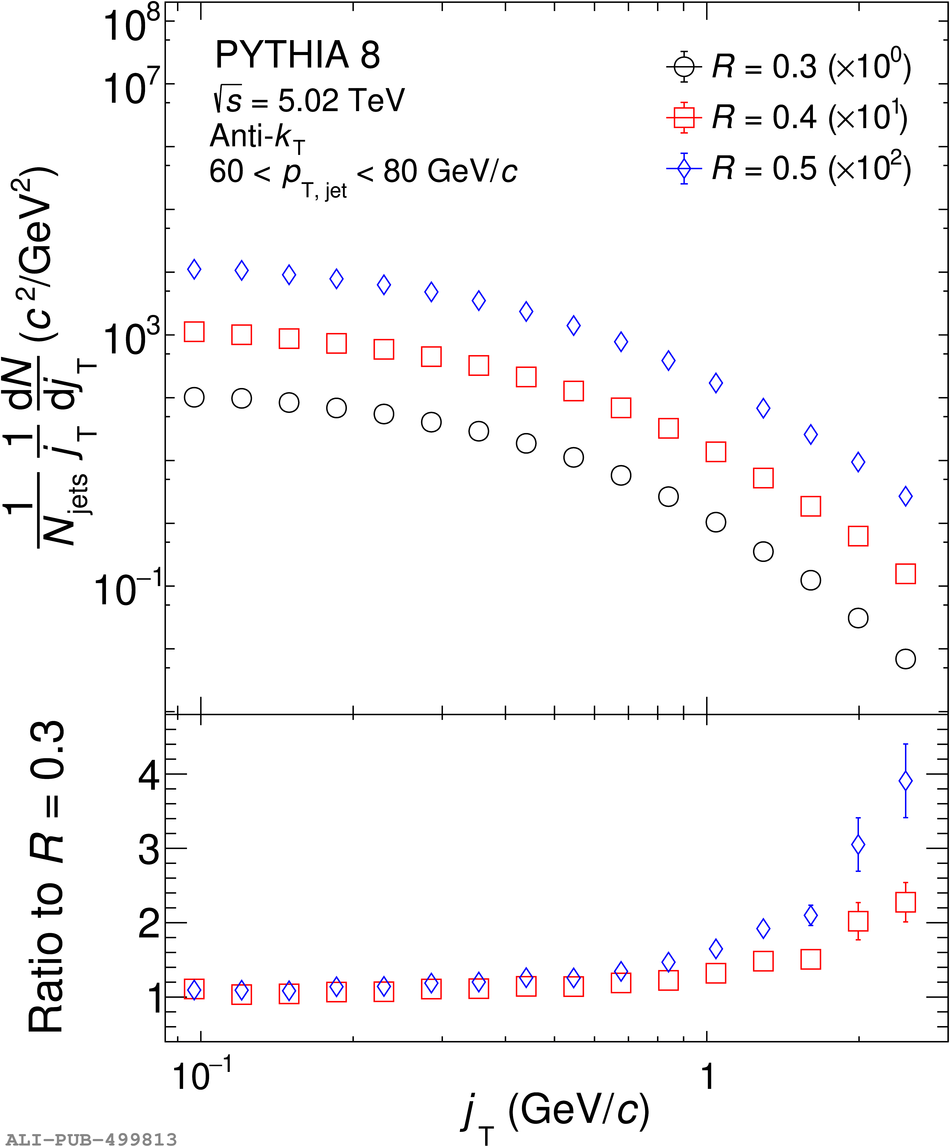# Figure 7

 The effect of changing the $R$ parameter in jet finding on $\jt$ distributions obtained with PYTHIA 8 simulations. Comparison of (a) $\jt$ signal distributions for different $R$ parameters and their ratios to that of $R=0.3$ and (b) RMS values of the wide and narrow components and their ratios to that of $R=0.3$ for the wide component only.